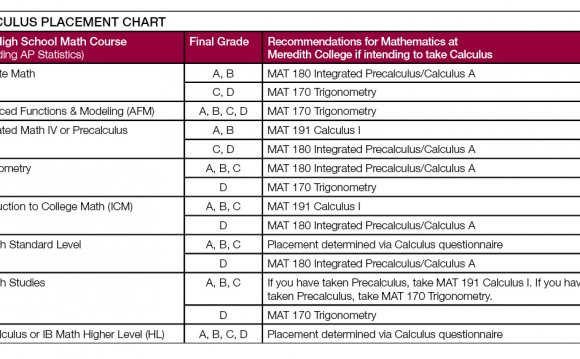# Applications of Mathematics in Computer Science

March 21, 2023Math is one of the foundations of computer sciences. It’s also one of the more crucial elements in computer sciences. So how is mathematics really applied in computer science? Despite advanced mathematics not applied frequently, basic mathematics, most importantly algebra, is the main ingredient for a successful computer scientist.
Many of the functions and operators in all programing languages require some knowledge in mathematics. For example, these operators include arithmetic, comparison, logical, assignment and conditional operators. All of the aforementioned tasks need mathematics for them to be used and properly applied, specially the arithmetic and conditional operators.
Computer sciences heavily rely on algorithms, which the latter in turn heavily relies on mathematics. ‘Theoretical computer science’ strongly involves discrete mathematics. Discrete mathematics is basically the study of mathematical structures that are discrete rather than continuous, and so this ‘theoretical’ branch of computer sciences involves a lot of mathematics, in the form of graphs, algorithms, computational geometry, quantum computation, algebra, computational number theory. In fact, the use of mathematics in computer sciences depends on the latter’s department. A beginner in programming may not need mathematics, but as the programmer advances through the level of difficulty, he / she will have to use more advanced mathematics.
Even though we don’t see any, computers operate using binary digits, which is basically mathematics. Physics plays a role in certain computer science branches. And since mathematics and physics are hugely related, this only provides us with more proof on how much mathematics is in computer sciences. More mathematical applications in computer science are sets, probability and Boolean algebra. Many applications such as calculators, video games and graphical applications are compelled to the use of mathematics. In my opinion, without proper mathematics, any computer scientist would be on the path to failure. For many programs to be created and written, logic is the central necessity, which in turn is mainly rooted to mathematics and mathematical principles. Hence, mathematics provides the right pathway towards success in computing.
‘Formal methods’ is mathematically based technique for the specification, verification and development of software and hardware systems. It is another branch in computer science that depends on mathematics some great extent, mostly algebraic manipulations and logic calculi.
So to conclude, mathematics is considered a necessity for any successful computer scientist. All computer science applications require mathematics to some degree. To tackle the most challenging of programs, mathematics will be of huge aid.

Questions:
1) How exactly is mathematics related to computer science?
2) How can mathematics help advance computer science?

Source: www.contrib.andrew.cmu.edu
##### INTERESTING VIDEOBilal Khan - Professor of Mathematics and Computer Science ...Components of Discretemathematics in Computer Science